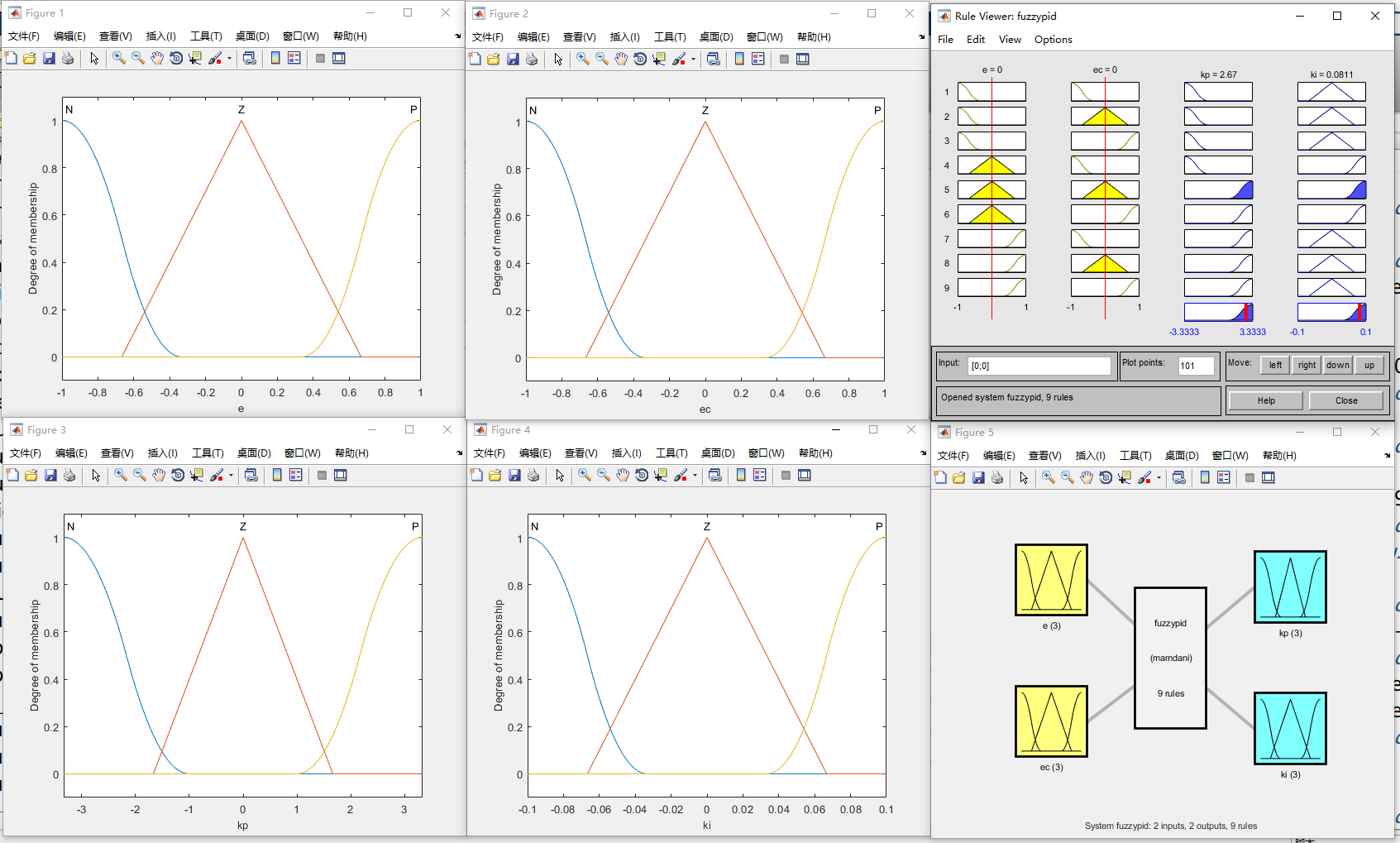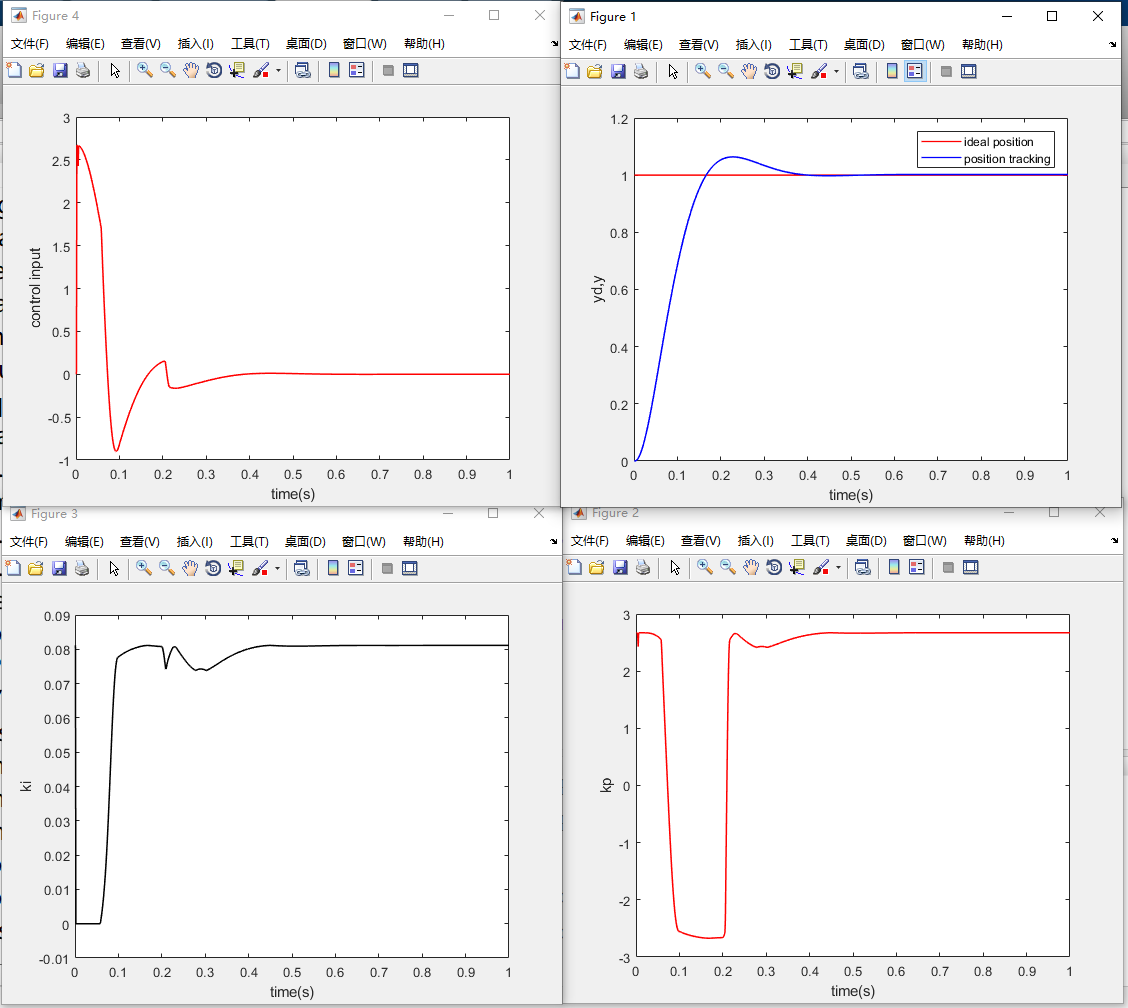• 模糊PD和模糊PI控制器，对于学习模糊控制的很有作用，还有PID的
• 下面介绍包含模糊PI控制器算法的DSP应用程序，下面的程序段就是数字模糊PI控制器的控制表程序，程序的文件名是Fuzzy_PI．asm，每行程序都有详细的解释说明，由于模糊PI控制器是在数字PID控制器的基础上改进的，又...
• 模糊PI控制器回馈好朋友的帮助帮助其他需要帮助的人-模糊PD控制器.rar 希望对大家有用，好朋友们matlab
• 为提高永磁同步电动机控制系统的抗扰性能，设计了一种新型的模糊PI控制器。该控制器包括模糊控制器和PI控制器，采用模糊开关选择器根据系统的状态实现不同控制器之间的切换，实现简单。将该新型控制器用于永磁同步...
• 基于模糊PI控制器的SRM直接转矩控制系统研究，李大鹏，蒯松岩，开关磁阻电机的直接转矩控制能够有效的把转矩脉动限制在一个小的误差滞环带内，降低了转矩脉动，但由于速度控制器采用PI调节器，�
• 针对网络所诱导的长时延,将Smith预估器应用到网络控制系统中,并通过与模糊PI控制方法相结合,构成Smith预估模糊PI控制器,设计出的控制器使闭环系统具有较好的性能和稳定性,并对于模型失配的情况具有较高的鲁棒性....
基于MATLAB的模糊pi控制器的设计
% 模糊规则隶属函数的建立
a=newfis(‘fuzzypid’);
% 添加第一个输入变量e
% 添加第二个输入变量ec
% 添加第一个输出变量kp
% 添加第二个输出变量ki
% 添加模糊规则
rulelist=[
1 1 1 2 1 1;
1 2 1 2 1 1;
1 3 1 2 1 1;
2 1 1 3 1 1;
2 2 3 3 1 1;
2 3 3 3 1 1;
3 1 3 2 1 1;
3 2 3 2 1 1;
3 3 3 2 1 1
];
% 将模糊规则加入到a中
% 解模糊
a=setfis(a,‘defuzzmethod’,‘centroid’);
% 保存到系统磁盘中
writefis(a,‘fuzzypid’);
% 读取模糊推理系统
% 作图
figure(1);
plotmf(a,‘input’,1);
figure(2);
plotmf(a,‘input’,2);
figure(3);
plotmf(a,‘output’,1);
figure(4);
plotmf(a,‘output’,2);
figure(5);
plotfis(a);
showrule(a)
ruleview fuzzypid;
% 模糊pid主控程序
% 加载模糊规则
% 建立控制对象的模型
ts=0.001;                      %设定步长
sys=tf(133,[1,25,0]);          %将连续型传递函数通过z变换为离散型
dsys=c2d(sys,ts,‘z’);          %离散化
[num,den]=tfdata(dsys,‘v’);    %获得离散建模的分子分母
% 设定系统相关参数的初值
u_1=0;u_2=0;
y_1=0;y_2=0;
e_1=0;ec_1=0;ei=0;
kp0=0;ki0=0;
for k=1:1:1000
time(k)=k*ts;    %设定步数
rin(k)=1;
k_pid=evalfis([e_1,ec_1],a);  %离散化后提取分子、分母
kp(k)=kp0+k_pid(1);
ki(k)=ki0+k_pid(2);
u(k)=kp(k)*e_1+ki(k)*ei;

yk=-den(2)*y_1-den(3)*y_2+num(2)*u_1+num(3)*u_2;
e(k)=rin(k)-y(k);

u_2=u_1;
u_1=u(k);
y_2=y_1;
y_1=y(k);

ei=ei+e(k)*ts;
ec(k)=(e(k)-e_1)/ts;

e_1=e(k);
ec_1=ec(k);

end
figure(1);
plot(time,rin,‘r’,time,y,‘b’,‘linewidth’,1);
xlabel(‘time(s)’);
ylabel(‘yd,y’);
legend(‘ideal position’,‘position tracking’);
figure(2);
plot(time,kp,‘r’,‘linewidth’,1);
xlabel(‘time(s)’);
ylabel(‘kp’);
figure(3);
plot(time,ki,‘k’,‘linewidth’,1);
xlabel(‘time(s)’);
ylabel(‘ki’);
figure(4);
plot(time,u,‘r’,‘linewidth’,1);
xlabel(‘time(s)’);
ylabel(‘control input’);
结果显示：展开全文matlab pid
• 设计模糊PI控制器后，做了一系列实验。图是电机在模糊PI控制下，不同设定速度的阶跃响应。其中，曲线a表示电机空载情况下的阶跃晌应；曲线b表示电机长时间运行后温度上升的情况下的阶跃响应；曲线c表示当电机加上3...
• 设计模糊PI控制器后，做了一系列实验。图是电机在模糊PI控制下，不同设定速度的阶跃响应。其中，曲线a表示电机空载情况下的阶跃晌应；曲线b表示电机长时间运行后温度上升的情况下的阶跃响应；曲线c表示当电机加上3...
• 使用模糊调节PI控制器的三区域系统负载频率控制。matlab
• 针对传统比例积分控制参数难以整定...当电流内环控制采用双环重复模糊PI控制器时,电源侧电流总谐波畸变率明显下降,总谐波畸变率为0.73%。该控制策略下所建立的理论模型与实际非理想模型更接近,谐波电流的补偿精度更高。
• 研究结果表明:改进遗传优化模糊PI控制器具有响应速度快,无超调等特性,鲁棒性和抗干扰能力强,电机动、静态性能得到明显改善.新型控制方法有效提高了无刷直流电机控制性能,并为无刷直流电机高性能控制的理论研究和实际...
• 提出了一种参数自整定模糊 PI控制器,用于永磁同步电机矢量控制系统,改善其动态性能。仿真 结果表明,加入模糊 PI控制的永磁同步电机矢量控制系统比 PI控制系统在转速、转矩方面的控制性能都有较大 的提高。
• 在该模型中，模糊逻辑控制 FLC 代替 PI 控制器来估计速度。matlab
• 自适应模糊PI控制，对风力发电领域的控制有更深的理解
• 该控制器通过改进三相线电压的正负序分量分解方法，并为模糊PI控制器设定合理的控制规则和合适的参数，使输出电压同时拥有稳定，三相平衡和响应快速的特点．利用一台三相逆变电源样机对该控制器和传统控制器进行对比...
• 为了解决高阶次、多变量、非线性、强耦合的直线二级倒立摆的稳定控制问题，设计了一种参数自校正模糊PI控制器算法。结合线性二次型最优调节器(LQR)方法，设计了融合函数，降低了模糊控制器的维数、减少了控制器规则...二级倒立摆
• 常规PI控制算法是工业控制领域中应用最广的一种算法，该算法简单，适应性强，具有一定的鲁棒性，能够满足一般生产...本文引入模糊PI控制器并将其应用到交流推进电机调速系统中去，期望改善调速系统的动态和稳态性能。
• 文中针对传统蓄电池充电时间长，具有过冲、欠冲、析气的问题,结合模糊控制...仿真结果表明,加入了模糊PI控制的系统具有更好的动态响应特性和稳态特性。自整定PI模糊控制器具有控制精度高,超调小，动态性能好的特点。
• 有源电力滤波器直流侧电压模糊PI控制，王超，，传统的直流侧电压控制方法采用PI控制，本文推导了被控对象的传函、设计了PI控制器的参数；在此基础上应用模糊理论设计了基于模糊PI
• 在分析无刷直流电机数学模型的基础上,将模糊控制与传统的 PID控制相结合,设计了...仿真实验表明,用模糊 PI控制器代替普通的 PI控制器,可以使 BLDC的整体性能得到显著改善,是高性能 BLDC调速系统开发的一个重要方向。
• 本文重点介绍了模糊自适应控制器和常规PI 控制器组成的模糊自适应PI 控制器的设计方法，并用这种方法设计了一个有源功率因数校正（APFC）系统。通过MATLAB 仿真表明，引入模糊PI 控制设计的APFC 系统具有响应快，...
• 行业分类-电子电器-基于双模糊PI控制的航空宽变频三级式电机调压方法.zip
• 求提出了路径的识别与轨迹跟踪算法，即分段模糊 ＰＩ控制算法，以适应 ＡＧＶ 小车在直行、转弯和停车三种状态下存在 的位置偏差和角度偏差的控制。运用 Ｍａｔｌａｂ软件中 Ｓｉｍｕｌｉｎｋ模块搭建模糊控制器的...
• 针对目前的发展趋势,以斜波信号和恒值信号作为信号源,介绍了模糊PI D的控制原理,提出了运用非对称隶属度函数方法解决该系统中存在的参数时变的问题,缩小了误差,提高了系统的响应能力。实验结果表明系统的随动灵敏度......